# Low Power Factor Wattmeter: What is it? (And Why is it Used)

## What is a Low Power Factor Wattmeter?

A low power factor meter is an instrument that is used to accurately measure low values of power factor. Before we study more about the low power factor meter, we need to understand why we need a low power factor wattmeter in the first place (as opposed to a standard electrodynamometer wattmeter)

The answer is simple: a standard wattmeter gives inaccurate results.

Now there are two main situations where we should not use an ordinary wattmeter in measuring a low power factor:

1. The value of deflecting torque is very low even though we fully excite the current and pressure coils.
2. Errors due to pressure coil inductance.

The above two reasons give very inaccurate results thus we should not use normal or ordinary watt meters in measuring the low value of the power factor.

However, by doing some modifications or adding some new features we can use a modified electrodynamic wattmeter or low power factor to measure the low power factor accurately.

Ideally, we would increase the power factor through power factor correction. But sometimes it is not possible to get the power factor higher enough (due to technical reasons or budget concerns).

Here we are going to discuss, where we need to do the modification. These are discussed one by one below:

(1) The electrical resistance of the ordinary wattmeter’s pressure coil is reduced to a low value such that current in the pressure coil circuit is increased, thus it leads to. In this category two cases, diagrams arise and these are shown below: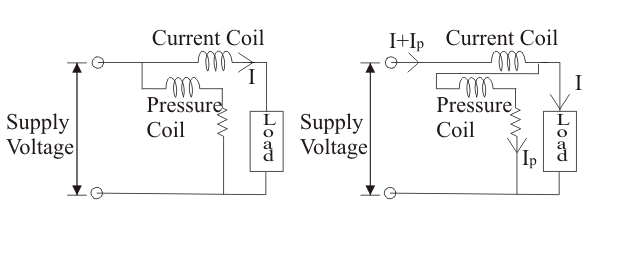In the first category both the ends of the pressure, the coil is connected to the supply-side (i.e. current coil is in series with the load). The supply voltage is equal to the voltage across the pressure coil. Thus in this case we have power shown by the first wattmeter is equal to the power loss in the load plus power loss in the current coil.In the second category, the current coil is not in series with the load and the voltage across the pressure coil is not equal to the applied voltage.

The voltage across the pressure coil is equal to the voltage across the load. This power shown by the second-watt meter is equal to the power loss in the load plus the power loss in the pressure coil.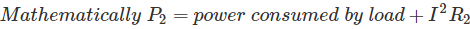From the above discussion, we conclude that in both cases we have some amount of errors hence there is a need to do some modification in the above circuits to have minimum error.

The modified circuit is shown below:
We have used here a special coil called compensating coil, it carries a current equal to the sum of two currents i.e load current plus pressure coil current.

The pressure coil is placed such that the field produced by the compensating coil is opposed by the field produced by the pressure coil as shown in the above circuit diagram.Thus the net field is due to the current I only. Hence in this way errors caused by pressure coil can be neutralized.

(2) We require compensating coil in the circuit in order to make the low power factor meter. It is the second modification that we have discussed in detail above.

(3) Now the third point deals with the compensation of the inductance of the pressure coil, which can be achieved by doing modification in the above circuit.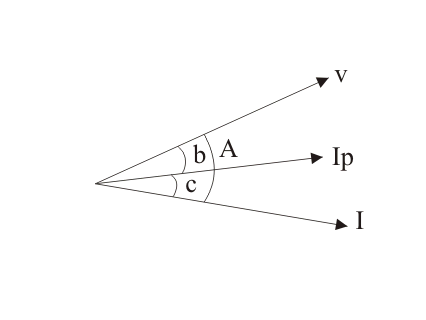Now let us derive an expression for the correction factor for pressure coil inductance. And from this correction factor, we are going to derive an expression for error due to the inductance of the pressure coil.

If we consider the inductance of the pressure coil we don’t have voltage across pressure in phase with the applied voltage.

Hence in that case it lags by an angle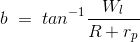Where, R is electrical resistance in series with pressure coil, rp is pressure coil resistance, here we also conclude that the current in the current coil is also lagging by some angle with the current in pressure coil. And this angle is given by C = A – b. At this time reading of the voltmeter is given by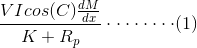Where, Rp is (rp+R) and x is angle. If we ignore the effect of the inductance of pressure i.e putting b = 0 we have expression for true power as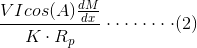On taking the ratio of equations (2) and (1) we have an expression for correction factor as written below: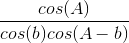And from this correction factor error can be calculated as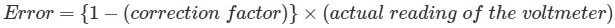On substituting the value of correction factor and taking suitable approximation we have an expression for error as VIsin(A)*tan(b).Now we know that the error caused by pressure coil inductance is given by the expression e = VIsin(A) tan(b), if the power factor is low (i.e in our case the value of φ is large hence we have a large error).

Thus in order to avoid this situation, we have connected the variable series resistance with a capacitor as shown in the above figure.

This final modified circuit so obtained is called the low power factor meter.

A modern low power factor meter is designed such that it gives high accuracy while measuring power factors even lower than 0.1.

Want To Learn Faster? 🎓
Get electrical articles delivered to your inbox every week.
No credit card required—it’s 100% free.